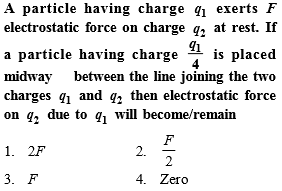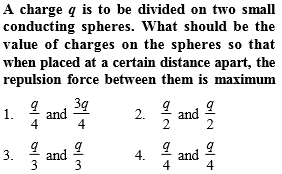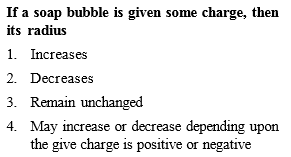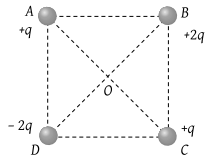Concept Videos :-

#2 | Basic Properties of Charge
#3 | Coulomb's Law Numericals
#4 | Dielectrical Constant
#5 | Principle of Superposition

Concept Questions :-

Coulomb's law
Explanation is a part of a Paid Course. To view Explanation Please buy the course.

Difficulty Level:Concept Videos :-

#2 | Basic Properties of Charge
#3 | Coulomb's Law Numericals
#4 | Dielectrical Constant
#5 | Principle of Superposition

Concept Questions :-

Coulomb's law
Explanation is a part of a Paid Course. To view Explanation Please buy the course.

Difficulty Level:Concept Videos :-

#2 | Basic Properties of Charge
#3 | Coulomb's Law Numericals
#4 | Dielectrical Constant
#5 | Principle of Superposition

Concept Questions :-

Coulomb's law
Explanation is a part of a Paid Course. To view Explanation Please buy the course.

Difficulty Level:

The law, governing the force between electric charges is known as

(1) Ampere's law

(2) Ohm's law

(4) Coulomb's law

Concept Videos :-

#2 | Basic Properties of Charge
#3 | Coulomb's Law Numericals
#4 | Dielectrical Constant
#5 | Principle of Superposition

Concept Questions :-

Coulomb's law
Explanation is a part of a Paid Course. To view Explanation Please buy the course.

Difficulty Level:

Fg and Fe represents gravitational and electrostatic force respectively between electrons situated at a distance 10 cm. The ratio of Fg/ Fe is of the order of

(1) 1042

(2) 10

(3) 1

(4) 10–43

Concept Videos :-

#2 | Basic Properties of Charge
#3 | Coulomb's Law Numericals
#4 | Dielectrical Constant
#5 | Principle of Superposition

Concept Questions :-

Coulomb's law
Explanation is a part of a Paid Course. To view Explanation Please buy the course.

Difficulty Level:

A soap bubble is given a negative charge, then its radius

(1) Decreases

(2) Increases

(3) Remains unchanged

(4) Nothing can be predicted as information is insufficient

Concept Videos :-

#2 | Basic Properties of Charge
#3 | Coulomb's Law Numericals
#4 | Dielectrical Constant
#5 | Principle of Superposition

Concept Questions :-

Coulomb's law
Explanation is a part of a Paid Course. To view Explanation Please buy the course.

Difficulty Level:

Four charges are arranged at the corners of a square ABCD, as shown in the adjoining figure. The force on the charge kept at the centre O is(1) Zero

(2) Along the diagonal AC

(3) Along the diagonal BD

(4) Perpendicular to side AB

Concept Videos :-

#2 | Basic Properties of Charge
#3 | Coulomb's Law Numericals
#4 | Dielectrical Constant
#5 | Principle of Superposition

Concept Questions :-

Coulomb's law
Explanation is a part of a Paid Course. To view Explanation Please buy the course.

Difficulty Level:

A total charge Q is broken in two parts Q1 and Q2 and they are placed at a distance R from each other. The maximum force of repulsion between them will occur, when

(1) ${Q}_{2}=\frac{Q}{R},\text{\hspace{0.17em}}{Q}_{1}=Q-\frac{Q}{R}$

(2) ${Q}_{2}=\frac{Q}{4},\text{\hspace{0.17em}}{Q}_{1}=Q-\frac{2Q}{3}$

(3) ${Q}_{2}=\frac{Q}{4},\text{\hspace{0.17em}}{Q}_{1}=\frac{3Q}{4}$

(4) ${Q}_{1}=\frac{Q}{2},\text{\hspace{0.17em}}{Q}_{2}=\frac{Q}{2}$

Concept Videos :-

#2 | Basic Properties of Charge
#3 | Coulomb's Law Numericals
#4 | Dielectrical Constant
#5 | Principle of Superposition

Concept Questions :-

Coulomb's law
Explanation is a part of a Paid Course. To view Explanation Please buy the course.

Difficulty Level:

Three charges 4q, Q and q are in a straight line in the position of 0, l/2 and l respectively. The resultant force on q will be zero, if Q =

(1) – q

(2) –2q

(3) $-\frac{q}{2}$

(4) 4q

Concept Videos :-

#2 | Basic Properties of Charge
#3 | Coulomb's Law Numericals
#4 | Dielectrical Constant
#5 | Principle of Superposition

Concept Questions :-

Coulomb's law
Explanation is a part of a Paid Course. To view Explanation Please buy the course.

Difficulty Level:

Two small spheres each having the charge +Q are suspended by insulating threads of length L from a hook. This arrangement is taken in space where there is no gravitational effect, then the angle between the two suspensions and the tension in each will be

(1) ${180}^{o},\text{\hspace{0.17em}}\frac{1}{4\pi {\epsilon }_{0}}\frac{{Q}^{2}}{{\left(2L\right)}^{2}}$

(2) ${90}^{o},\text{\hspace{0.17em}}\frac{1}{4\pi {\epsilon }_{0}}\frac{{Q}^{2}}{{L}^{2}}$

(3) ${180}^{o},\text{\hspace{0.17em}}\frac{1}{4\pi {\epsilon }_{0}}\frac{{Q}^{2}}{2{L}^{2}}$

(4) ${180}^{o},\text{\hspace{0.17em}}\frac{1}{4\pi {\epsilon }_{0}}\frac{{Q}^{2}}{{L}^{2}}$

Concept Videos :-

#2 | Basic Properties of Charge
#3 | Coulomb's Law Numericals
#4 | Dielectrical Constant
#5 | Principle of Superposition

Concept Questions :-

Coulomb's law
Explanation is a part of a Paid Course. To view Explanation Please buy the course.

Difficulty Level: